Algebraic Simplification

In the following examples and problems, the term "simplify" indicates to eliminate compound fractions, factor as much as possible, put terms over a common denominator when feasible, and avoid negative exponents.

Ex 1 Simplify the expression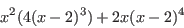.

Sol.

Ex 2 Simplify the expression.

Sol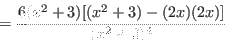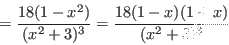.

Pr A Simplify the expression.

Pr B Simplify the expression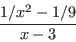.

Pr C Simplify the expressionby eliminating the radicals in the numerator.

Pr 1 Simplify the expression.

Pr 2 Simplify the expression.

Pr 3 Simplify the expression.

Pr 4 Simplify the expression.

Pr 5 Simplify the expression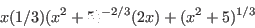.

Pr 6 Simplify the expression.

Pr 7 Simplify the expression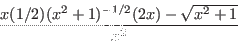.

Pr 8 Simplify the expression.
Lawrence Marx 2012-08-13